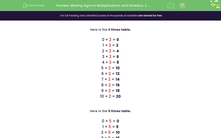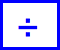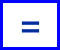# Use the Correct Signs to Create Multiplication and Division Statements

In this worksheet, students will complete multiplication and division statements by selecting the missing sign.Key stage:  KS 1

Curriculum topic:   Number: Multiplication and Division

Curriculum subtopic:   Calculate Using Multiplication/Division For Known Tables

Popular topics:   Times Table worksheets

Difficulty level:#### Worksheet Overview

In this activity, we are going to be completing multiplication and division sentences.

Here is the 2 times table.

0 × 2 = 0

1 × 2 = 2

2 × 2 = 4

3 × 2 = 6

4 × 2 = 8

5 × 2 = 10

6 × 2 = 12

7 × 2 = 14

8 × 2 = 16

9 × 2 = 18

10 × 2 = 20

Here is the 5 times table.

0 × 5 = 0

1 × 5 = 5

2 × 5 = 10

3 × 5 = 15

4 × 5 = 20

5 × 5 = 25

6 × 5 = 30

7 × 5 = 35

8 × 5 = 40

9 × 5 = 45

10 × 5 = 50

Here is the 10 times table.

0 × 10 = 0

1 × 10 = 10

2 × 10 = 20

3 × 10 = 30

4 × 10 = 40

5 × 10 = 50

6 × 10 = 60

7 × 10 = 70

8 × 10 = 80

9 × 10 = 90

10 × 10 = 100

Example 1

Fill in the missing symbol  ×,  ÷  or  =

205  =  4

What is the connection in the times tables between 20, 5 and 4 ?

You should know that   4 × 5 = 20

So...

205  =  4

Example 2

Fill in the missing symbol  ×,  ÷  or  =

189  ×  2

What is the connection in the times tables between 18, 9 and 2 ?

You should know that   9 × 2 = 18

So...

189  ×  2

Shall we have a go at some questions now and see if you're a maths superhero?### What is EdPlace?

We're your National Curriculum aligned online education content provider helping each child succeed in English, maths and science from year 1 to GCSE. With an EdPlace account you’ll be able to track and measure progress, helping each child achieve their best. We build confidence and attainment by personalising each child’s learning at a level that suits them.

Get started